### 3.5.7 Floating Point Types

(1)
For floating point types, the error bound is specified as a relative precision by giving the required minimum number of significant decimal digits.
##### Syntax
```(2)
floating_point_definition ::=
digits static_expression [real_range_specification]
(3)
real_range_specification ::=
range static_simple_expression .. static_simple_expression
```
##### Name Resolution Rules
(4)
The requested decimal precision, which is the minimum number of significant decimal digits required for the floating point type, is specified by the value of the expression given after the reserved word digits. This expression is expected to be of any integer type.
(5)
Each simple_expression of a real_range_specification is expected to be of any real type; the types need not be the same.
##### Legality Rules
(6)
The requested decimal precision shall be specified by a static expression whose value is positive and no greater than System.Max_Base_Digits. Each simple_expression of a real_range_specification shall also be static. If the real_range_specification is omitted, the requested decimal precision shall be no greater than System.Max_Digits.
(7)
A floating_point_definition is illegal if the implementation does not support a floating point type that satisfies the requested decimal precision and range.
##### Static Semantics
(8)
The set of values for a floating point type is the (infinite) set of rational numbers. The machine numbers of a floating point type are the values of the type that can be represented exactly in every unconstrained variable of the type. The base range (see 3.5) of a floating point type is symmetric around zero, except that it can include some extra negative values in some implementations.
(9)
The base decimal precision of a floating point type is the number of decimal digits of precision representable in objects of the type. The safe range of a floating point type is that part of its base range for which the accuracy corresponding to the base decimal precision is preserved by all predefined operations.
(10)
A floating_point_definition defines a floating point type whose base decimal precision is no less than the requested decimal precision. If a real_range_specification is given, the safe range of the floating point type (and hence, also its base range) includes at least the values of the simple expressions given in the real_range_specification. If a real_range_specification is not given, the safe (and base) range of the type includes at least the values of the range -10.0**(4*D) .. +10.0**(4*D) where D is the requested decimal precision. The safe range might include other values as well. The attributes Safe_First and Safe_Last give the actual bounds of the safe range.
(11)
A floating_point_definition also defines a first subtype of the type. If a real_range_specification is given, then the subtype is constrained to a range whose bounds are given by a conversion of the values of the simple_expressions of the real_range_specification to the type being defined. Otherwise, the subtype is unconstrained.
(12)
There is a predefined, unconstrained, floating point subtype named Float, declared in the visible part of package Standard.
##### Dynamic Semantics
(13)
The elaboration of a floating_point_definition creates the floating point type and its first subtype.
##### Implementation Requirements
(14)
In an implementation that supports floating point types with 6 or more digits of precision, the requested decimal precision for Float shall be at least 6.
(15)
If Long_Float is predefined for an implementation, then its requested decimal precision shall be at least 11.
##### Implementation Permissions
(16)
An implementation is allowed to provide additional predefined floating point types, declared in the visible part of Standard, whose (unconstrained) first subtypes have names of the form Short_Float, Long_Float, Short_Short_Float, Long_Long_Float, etc. Different predefined floating point types are allowed to have the same base decimal precision. However, the precision of Float should be no greater than that of Long_Float. Similarly, the precision of Short_Float (if provided) should be no greater than Float. Corresponding recommendations apply to any other predefined floating point types. There need not be a named floating point type corresponding to each distinct base decimal precision supported by an implementation.
(17)
An implementation should support Long_Float in addition to Float if the target machine supports 11 or more digits of precision. No other named floating point subtypes are recommended for package Standard. Instead, appropriate named floating point subtypes should be provided in the library package Interfaces (see B.2).

NOTES
(18)
(33) If a floating point subtype is unconstrained, then assignments to variables of the subtype involve only Overflow_Checks, never Range_Checks.
##### Examples
(19)
Examples of floating point types and subtypes:
```(20)
type Coefficient is digits 10 range -1.0 .. 1.0;
(21)
type Real is digits 8;
type Mass is digits 7 range 0.0 .. 1.0E35;
(22)
subtype Probability is Real range 0.0 .. 1.0;   --   a subtype with a smaller range
```

####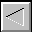|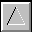|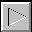|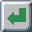|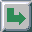TOC -- / --.-- / --.--.-- | Index | Search | Syntax | Help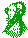-- Email comments, additions, corrections, gripes, kudos, etc. to:

Magnus Kempe -- Magnus.Kempe@di.epfl.ch